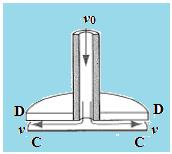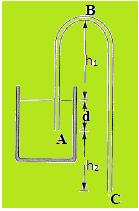Courses

# Notes | EduRev

## JEE Revision Notes

Created by: Learners Habitat

## JEE : Notes | EduRev

The document Notes | EduRev is a part of the JEE Course JEE Revision Notes.
All you need of JEE at this link: JEE

Question 1: Water is pumped steadily out of a flooded basement at a speed of 5.30 m/s through a uniform hose of radius 9.70 mm. The hose passes out through a window 2.90 m above the water line. How much power is supplied by the pump?
Concept:-
The kinetic energy of the water per unit mass when it leaves from the uniform hose through the window is
K = ½ v2
Here, mass of the flow of water is  and speed of the water flow is v.
The corresponding potential energy per unit mass of the flow of water through the window is
U = gh
Here, acceleration due to gravity of the Earth is g and height of the window from the basement is h.
The volume rate of the flow of water from the hose through the window is
R = vA
Here, cross-sectional area of the hose is A and speed of the water flow is v.
The mass rate of the flow of water is
Rm = ρR
Here, density of the water is ρ.
The power supplied by the pump is given by
P = (K+U) Rm
Solution:-
The cross-sectional area of the hose is
A = πr2
Here, radius of the hose is r.
Insert the values of  in the equation R = vA  gives
R = v (πr2)
Using the above values in the equation Rm = ρR
gives
Rm = ρv (πr2)
Substitute the values of K, U and Rm in the equation P = (K+U) Rm gives
P = [(1/2) v2 + gh] [ρv (πr2)]
To obtain the power supplied by the pump, substitute 5.30 m/s  for v, 9.8 m/s2 for g, 2.90 m  for h, 1000 kg/m3 for ρ and 9.70 mm for r in the above equation gives
P = [(1/2) v2 + gh] [ρv (πr2)]
= [(1/2) (5.30 m/s)2 + (9.8 m/s)2 (2.90 m)] [(1000 kg/m3) (5.30 m/s) (3.14) (9.70 mm)2 (10-3 m/1 mm)2]
= (14.045 m2/s2 + 28.42 m2/s2) (1.56584578 kg/s)
= (66.494 kg.m2/s) [1 W/(1kg.m2/s)]
= 66.494 W
Rounding off to three significant figures, the power supplied by the pump is 66.5 W.

Question 2: A hollow tube has a disk DD attached to its end as shown in the below figure. When air of density ρ is blown through the tube, the disk attracts the card CC. Let the area of the card be A and let v be the average air speed between the card and the disk. Calculate the resultant upward force on CC. Neglect the card’s weight; assume that v0<<v, where v0 is the air speed in the hollow tube.Concept:-
According to Bernoulli’s equation, the total energy of the steady flow of air in the hollow tube remains constant.
It is given as
p+ ½ ρv+ ρgh = constant
Here, pressure of the flow of air is p, density of the air is ρ, speed of the air in the hollow tube is v, acceleration due to gravity of the earth at the point of observation is g and position of the flow of air from the ground is h.
The resultant upward force on the card CC is equal to the difference in the pressure times the area of the card CC.
Solution:-
Apply Bernoulli’s equation just before it leaves the hollow tube, the total energy of the flow of air is
p1+ ½ ρv0+ ρgy1 = constant
Here, pressure of the flow of air just before it leaves the hollow tube is p1, speed of the air in the hollow tube is v0, and position of the flow of air from the ground before the air leaves the hollow tube is y1.
On the upper surface of the card CC, the total energy of the flow of air is
p2+ ½ ρv2 + ρgy2 = constant
Here, pressure of the flow of air above the car CC is p2, speed of the air above the card CC is v, and position of the flow of air above the card CC from the ground is y2.
The total energy for the flow of air remains the same when it flows from the hollow tube to the card CC.
Thus,
p1+ ½ ρv02 + ρgy= p2+ ½ ρv2 + ρgy2
The position of the flow of air is same for the same flow of air.
Thus,
y1 = y2
Also, it is given that v0<<v.
So, the speed in the hollow tube v0 can be neglected.
Insert y1 = y2   and neglecting v0 in the equation  p1+ ½ ρv02 + ρgy1 = p2+ ½ ρv2 + ρgy2
Gives
p1+ ½ ρv02 + ρgy1 = p2+ ½ ρv+ ρgy2
p1 - p2 = ½ ρv2
Now, the resultant upward force on the card CC is
F = (p1-p2) A
Substitute ½ ρv2 for p1-p2 in the above equation gives
F = (p1-p2) A
= ½ ρv2A
Therefore, the resultant upward force on the CC is ½ ρv2A.

Question 3: A siphon is a device for removing liquid from a container that is not to be tipped. It operates as shown in below figure. The tube must initially be filled, but once this has been done the liquid will flow until its level drops below the tube opening at A. The liquid has density ρ and negligible viscosity. (a) With what speed does the liquid emerge from the tube at C? (b) What is the pressure in the liquid at the topmost point B? (c) What is the greatest possible height h that a siphon may lift water?Concept:-
From Torricelli’s law, the speed of the water emerges from a depth h through a hole in a tank is
v =√2gh
Here, acceleration due to gravity is g.
The pressure at the depth h is
p = p0+ρgh
Here, pressure at the surface of the water is p0 and density of the water is ρ.
Solution:-
(a) Water will flow in the pipe and emerges from the point C. It is considered that water flows from depth equal to the d+h2.
Here, the equivalent depth of the water is
h = d+ h2
From equation v =√2gh, the speed of the water is
v =√2gh
=√2g(d+h2)
Therefore, the speed of the water emerges from the point C is √2g(d+h2).
(b) The pressure at the point C is
pC = ρg (h1+d+h2)
Now, the pressure at the point B is
pB = p0 – pC
Insert p= ρg (h1+d+h2) in the above equation gives
pB = p0 – pC= p- ρg (h1+d+h2)
Therefore, the pressure at the point B is p0 - ρg (h1+d+h2).
(c) Now, the maximum possible height is obtained as
p= ρgh
h = p0/ρg
Substitute 1.01×105 kg/m.s2 for p0, 1000 kg/m3 for ρ and 9.8 m/s2 for g in   the above equation gives
h = p0/ρg
= (1.01×105 kg/m.s2)/( 1000 kg/m3) (9.8 m/s2)
= 10.306 m
Rounding off to three significant figures, the greatest possible height that the siphon may lift water is 10.3 m

Offer running on EduRev: Apply code STAYHOME200 to get INR 200 off on our premium plan EduRev Infinity!

,

,

,

,

,

,

,

,

,

,

,

,

,

,

,

,

,

,

,

,

,

;